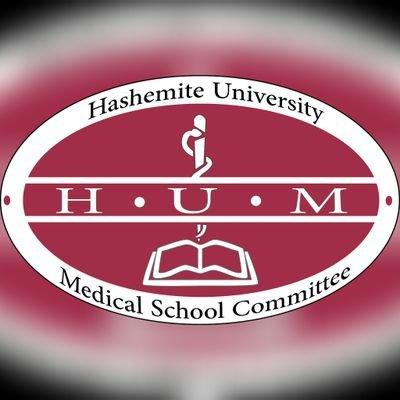# Biology 101 (Chapter 4 - Chapter 5) First Exam

42 Questions | Total Attempts: 570SettingsCreate your own QuizWelcome To Biology 101 Exam Be careful and focus The exam is easy :) GooD Luck

• 1.
All of these are true about " electron configuration" EXCEPT:
• A.

It determines the kind of bonds that an atom will form with other atoms

• B.

It determines the number of bonds that an atom will form with other atoms

• C.

It's the key to an atom's chemical characteristics

• D.

It determines the number of valence electrons

• E.

None of the above

• 2.
One of these is not true about an Carbon atom:
• A.

It has 4 valance electrons

• B.

It has 6 electrons

• C.

It can bound to another carbon atom

• D.

It forms hydrogen bounds by sharing it's 4 electrons

• E.

Two of the above

• 3.
When a Carbon forms 4 single bounds the arrangement of these bounds will be:
• A.

At the same plane

• B.

Tetrahedral shape with 109.5 bond angles

• C.

Tetrahedral shape with 105.9 bond angles

• D.

Two of the above

• E.

None of the above

• 4.
One of theses isn't a frequent bonding partner of Carbon:
• A.

Hydrogen

• B.

Sulfur

• C.

Nitrogen

• D.

Oxygen

• E.

Two of the above

• 5.
Carbon dioxide is often considered inorganic because:
• A.

It's a very simple molecule

• B.

It lacks nitrogen

• C.

It lacks hydrogen

• D.

It doesn't have single bonds

• E.

Two of the above

• 6.
Carbon can make organic compounds that vary in:
• A.

Length of carbon chains

• B.

Shape

• C.

Number of double bonds

• D.

Location of double bonds

• E.

All of the above

• 7.
Carbon chains Could have all of these forms(shapes) except:
• A.

Open(straight) chain

• B.

Ring chain

• C.

Random chain

• D.

Branched chain

• E.

None of the above

• 8.
The major components of petroleum are:
• A.

Enantiomers

• B.

Hydrocarbons

• C.

Amino acids

• D.

None of the above

• 9.
All of these are characteristics of hydrocarbons(such as fats and gasoline) except:
• A.

Dissolve in water

• B.

Hydrophobic compounds (because the great majority of their bonds are nonpolar carbon to hydrogen linkages)

• C.

Can undergo reactions that release a relatively large amount of energy

• D.

Two of the above

• E.

None of the above

• 10.
Compounds that have the same numbers of atoms of the same elements (same molecular formula) but different structures , is the definition of:
• A.

Structural isomers

• B.

Cis-trans isomers

• C.

Enantiomers

• D.

All of the above

• 11.
The two compounds in that figure are related as:
• A.

Geometric isomers

• B.

Strucural isomers

• C.

Enantiomers

• D.

None of the above

• 12.
One of these statements is TRUE about that figure:
• A.

It can make cis-trans isomers

• B.

It can make structural isomers

• C.

It can make enantiomers and asymmetric carbons are (b&c)

• D.

It can make enantiomers and asymmetric carbon is (b)

• E.

Two of the above

• 13.
Organic compounds with a sulfhydryl group are known as:
• A.

Alcohols

• B.

Organic acids

• C.

Amines

• D.

Thiols

• E.

Sulfylated compounds

• 14.
One of these statements is false about "Acetone":
• A.

Structural isomer with Propanal

• B.

Simplest ketone

• C.

Has a carboxyl group within the carbon skeleton

• D.

Consist of 3 carbons

• E.

None of the above

• 15.
Amino group is characterized by:
• A.

Charged by (-1) in ionized form

• B.

Acts as an acid

• C.

Can pick up H+ from the water in living organisms

• D.

Includes nitrogen atom

• E.

Two of the above

• 16.
If a polymer with 741 monomers is broken by hydrolysis , this will give us :
• A.

740 monomers

• B.

740 water molecules

• C.

741 water molecules

• D.

None of the above

• 17.
Carbohydrates macromolecules are:
• A.

Polymers called disaccharides composed of many monosaccharides joined by covalent bonds

• B.

Polymers called polysaccharides composed of many monosaccharides joined by hydrogen bonds

• C.

Polymers called polysaccharides composed of many monosaccharides joined by glycosidic linkages

• D.

None of the above

• 18.
The one true about relation between Glucose and Fructose is:
• A.

Both are aldoses

• B.

Structural isomers

• C.

They differ in molecular formula

• D.

Both are hexoses

• E.

Two of the above

• 19.
One of these is an example of a disaccharides:
• A.

Starch

• B.

Amylose

• C.

Galactose

• D.

Lactose

• 20.
Starch is found in planet cells in a structures called "plastids"
• A.

True

• B.

False

• 21.
The best describes the relation between amylose and amylopectin is:
• A.

Both are synthesized in animal cells

• B.

Both are branched

• C.

Both have 1-4 glycosidic linkages and 1-6 glycosidic linkages

• D.

Potato tubers and grains are the major sources of them

• E.

Two of the above

• 22.
Starch and cellulose differ in:
• A.

Function

• B.

• C.

Three-dimensional shape

• D.

Two of the above

• E.

All of the above

• 23.
β glucose mean that OH group attached to second carbon are above the plane of the glucose ring
• A.

True

• B.

False

• 24.
Cow harbors can digest cellulose by it's enzymes
• A.

True

• B.

False

• 25.
"insoluble fiber" refers mainly to:
• A.

Chitin

• B.

Cytoskeleton

• C.

Cellulose

• D.

None of the aboveBack to top
×

Wait!
Here's an interesting quiz for you.